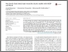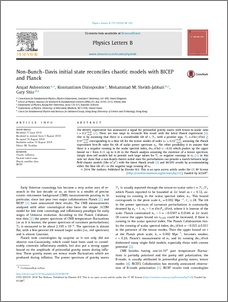# Non-bunch-Davis initial state reconciles chaotic models with BICEP and Planck

Ashoorioon, Amjad and Dimopoulos, Konstantinos and M. Sheikh-Jabbari, M. and Shiu, Gary (2014) Non-bunch-Davis initial state reconciles chaotic models with BICEP and Planck. Physics Letters B, 737. pp. 98-102. ISSN 0370-2693Preview
PDF (1-s2.0-S0370269314006054-main)
1_s2.0_S0370269314006054_main.pdf - Published Version

## Abstract

The BICEP2 experiment has announced a signal for primordial gravity waves with tensor-to-scalar ratio $r=0.2^{+0.07}_{-0.05}$ [arXiv:1403.3985]. There are two ways to reconcile this result with the latest Planck experiment [arXiv:1303.5082]. One is by assuming that there is a considerable tilt of $r$, $\mathcal{T}_r$, with a positive sign, $\mathcal{T}_r=d\ln r/d\ln k\gtrsim 0.57^{+0.29}_{-0.27}$ corresponding to a blue tilt for the tensor modes of order $n_T\simeq0.53 ^{+0.29}_{-0.27}$, assuming the Planck experiment best-fit value for tilt of scalar power spectrum $n_S$. The other possibility is to assume that there is a negative running in the scalar spectral index, $dn_S/d\ln k\simeq -0.02$ which pushes up the upper bound on $r$ from $0.11$ up to $0.26$ in the Planck analysis assuming the existence of a tensor spectrum. Simple slow-roll models fail to provide such large values for $\mathcal{T}_r$ or negative runnings in $n_S$ [arXiv:1403.3985]. In this note we show that a non-Bunch-Davis initial state for perturbations can provide a match between large field chaotic models (like $m^2\phi^2$) with the latest Planck result [arXiv:1306.4914] and BICEP2 results by accommodating either the blue tilt of $r$ or the negative large running of $n_S$.

Item Type:
Journal Article
Journal or Publication Title:
Physics Letters B
Uncontrolled Keywords:
/dk/atira/pure/subjectarea/asjc/3100/3106
Subjects:
Departments:
ID Code:
69499
Deposited By:
Deposited On:
27 May 2014 08:48
Refereed?:
Yes
Published?:
Published